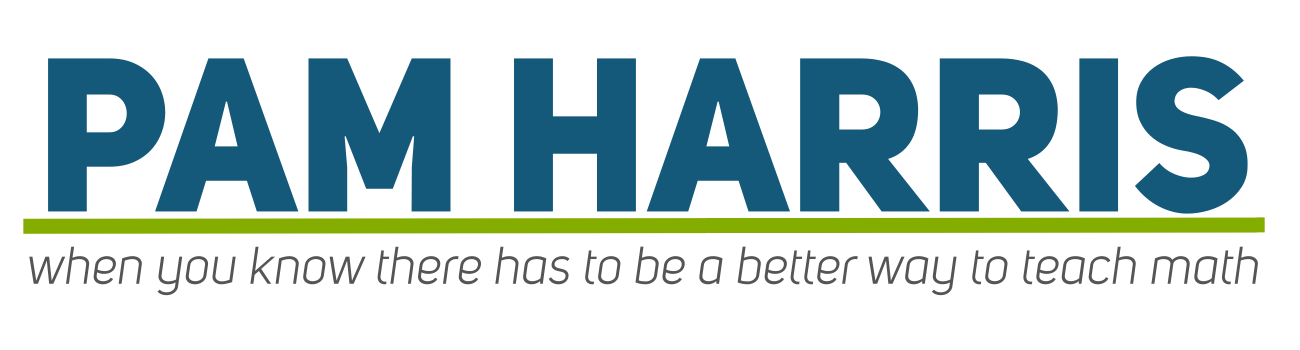Want students to know the single-digit multiplication facts through relationships?

✔ Use the power of Problem Strings. Get three Problem Strings that leverage problem order to develop powerful strategies.

Help students learn most missed facts. Build multiplicative relationships using facts students already know.

✔ Build multiplicative reasoning. Help students embrace the idea that multiplication facts are figure-out-able!

Want students to know the single-digit multiplication facts through relationships?

✔ Use the power of problem strings.

Get three Problem Stings that leverage problem order to develop powerful strategies.

✔ Help students learn most missed facts.

Build multiplicative relationships using facts students already know.

Build Multiplicative reasoning.

Help students embrace the idea that multiplication facts are figure-out-able!